# HTML5 Canvas ：碰撞检测

### 外接图形判别法

• 轴对称包围盒（Axis-Aligned Bounding Box），即无旋转矩形。
• 圆形碰撞
• 圆形与矩形（无旋转）
• 圆形与旋转矩形（以矩形中心点为旋转轴）

### 其他

• 地图格子划分
• 像素检测

See the Pen Boundary collision detection by Jc (@JChehe) on CodePen.

``````if(ball.left < 0 || ball.right  > rect.width)  ball.velocityX = -ball.velocityX
if(ball.top  < 0 || ball.bottom > rect.height) ball.velocityY = -ball.velocityY
``````

## 外接图形判别法

### 轴对称包围盒（Axis-Aligned Bounding Box）

``````rect1.x < rect2.x + rect2.width &&
rect1.x + rect1.width > rect2.x &&
rect1.y < rect2.y + rect2.height &&
rect1.height + rect1.y > rect2.y
``````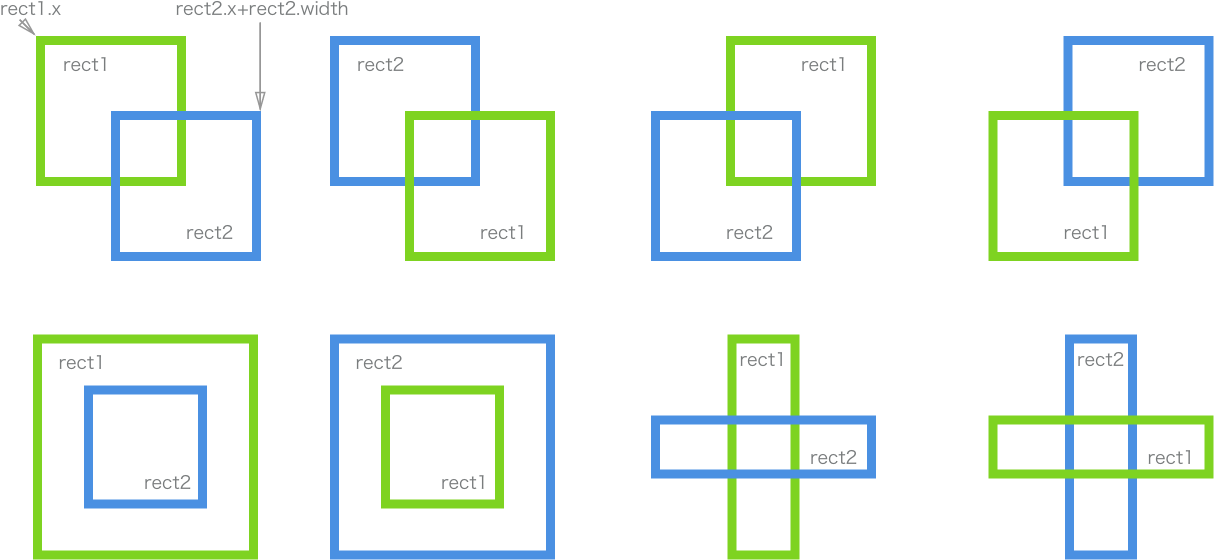See the Pen AxisAlignedBoundingBox collision detection by Jc (@JChehe) on CodePen.

• 相对局限：两物体必须是矩形，且均不允许旋转（即关于水平和垂直方向上对称）。
• 对于包含着图案（非填满整个矩形）的矩形进行碰撞检测，可能存在精度不足的问题。
• 物体运动速度过快时，可能会在相邻两动画帧之间快速穿越，导致忽略了本应碰撞的事件发生。

• （类）矩形物体间的碰撞。

### 圆形碰撞（Circle Collision）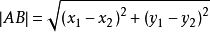``````Math.sqrt(
Math.pow(circleA.x - circleB.x, 2) + Math.pow(circleA.y - circleB.y, 2)
``````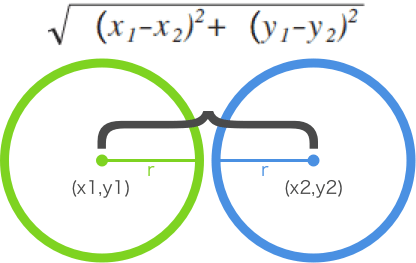See the Pen EZrorG by Jc (@JChehe) on CodePen.

• 与『轴对称包围盒』类似

• （类）圆形的物体，如各种球类碰撞。

### 圆形与矩形（无旋转）

`````` 矩形上离圆心最近的点为变量：closestPoint = {x, y};
矩形 rect = {x, y, w, h}; // 左上角与宽高
圆形 circle = {x, y, r}; // 圆心与半径
``````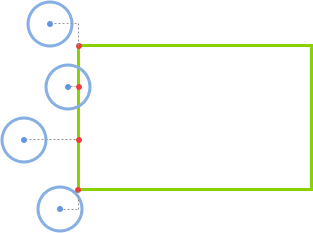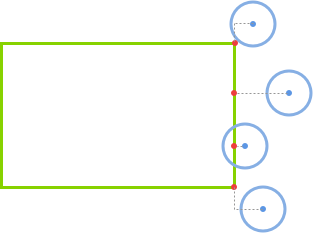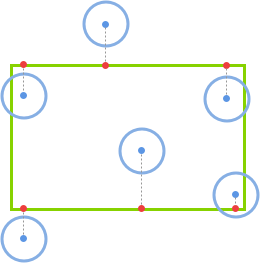``````var distance = Math.sqrt(Math.pow(closestPoint.x - circle.x, 2) + Math.pow(closestPoint.y - circle.y, 2))

if(distance < circle.r) return true // 发生碰撞
else return false // 未发生碰撞
``````

See the Pen Circle and Rectangle by Jc (@JChehe) on CodePen.

• 矩形需是轴对称的，即不能旋转。

### 圆形与旋转矩形（以矩形中心为旋转轴）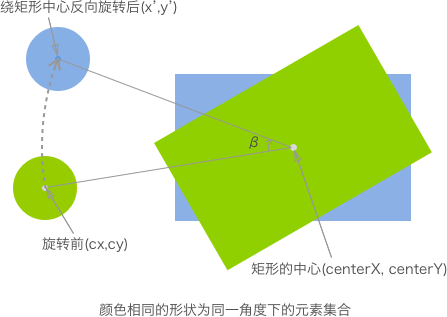``````x’ = cos(β) * (cx – centerX) – sin(β) * (cy – centerY) + centerX
y’ = sin(β) * (cx – centerX) + cos(β) * (cy – centerY) + centerY
``````

See the Pen Circle and Rotated Rectangle Collision Detection by Jc (@JChehe) on CodePen.

• 相对于圆形与矩形（未旋转）的方法，适用范围更广。

## 其他

### 地图格子划分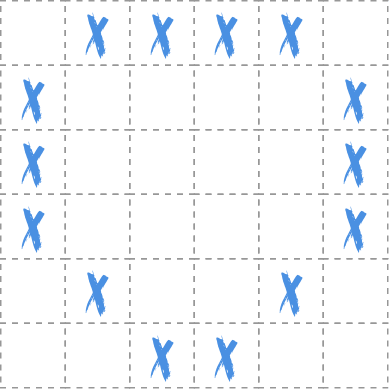``````// 通过特定标识指定（非）可行区域
map = [
[0, 0, 1, 1, 1, 0, 0, 0, 0],
[0, 1, 1, 0, 0, 1, 0, 0, 0],
[0, 1, 0, 0, 0, 0, 1, 0, 0],
[0, 1, 0, 0, 0, 0, 1, 0, 0],
[0, 1, 1, 1, 1, 1, 1, 0, 0]
],
// 设定角色的初始位置
player = {left: 2, top: 2}

// 移动前（后）判断角色的下一步的动作（如不能前行）
...
``````

See the Pen map cell collision detection by Jc (@JChehe) on CodePen.

• 适用场景局限。

• 推箱子、踩地雷等

### 像素检测

1. 如下述的案例中，通过将两个物体在 offscreen canvas 中判断同一位置（坐标）下是否同时存在非透明的像素。

2. 利用 canvas 的 globalCompositeOperation = 'destination-in' 属性。该属性会让两者的重叠部分会被保留，其余区域都变成透明。因此，若存在非透明像素，则为碰撞。

offscreen canvas：与之相关的是 offscreen rendering。正如其名，它会在某个地方进行渲染，但不是屏幕。“某个地方”其实是内存。渲染到内存比渲染到屏幕更快。—— Offscreen Rendering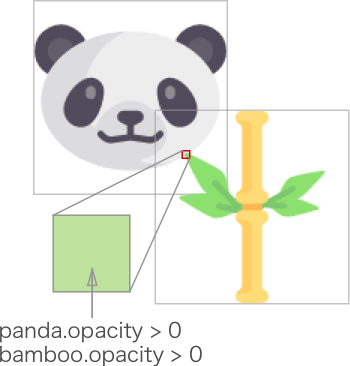See the Pen pixel collision detection by Jc (@JChehe) on CodePen.

• 因为需要检查每一像素来判定是否碰撞，性能要求比较高。

• 需要以像素级别检测物体是否碰撞。

## 光线投射法（Ray Casting）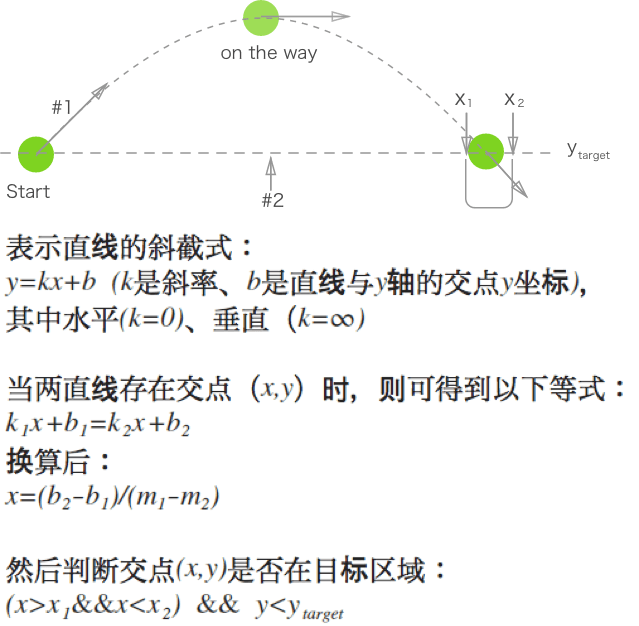• 两直线交点在桶口的左右边沿间
• 小球位于第二条线（#2）下方

See the Pen ray casting collision detection by Jc (@JChehe) on CodePen.

• 适合运动速度快的物体

• 适用范围相对局限。

• 抛球运动进桶。

## 分离轴定理（Separating Axis Theorem）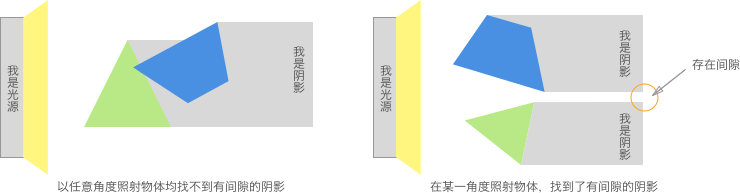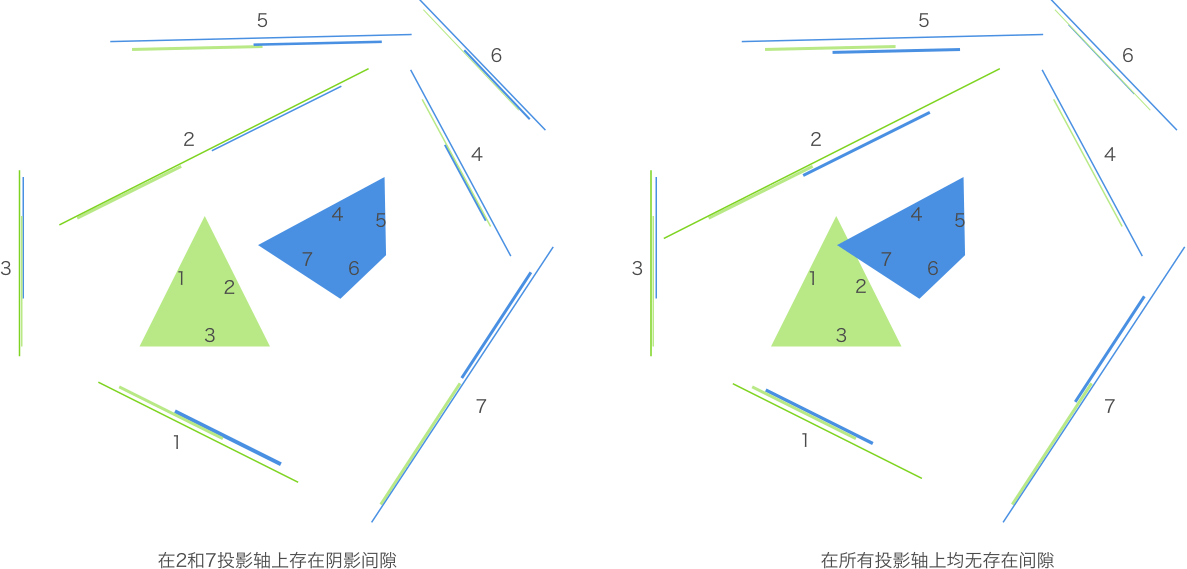``````function polygonsCollide(polygon1, polygon2) {
var axes, projection1, projection2

// 根据多边形获取所有投影轴
axes = polygon1.getAxes()
axes.push(polygon2.getAxes())

// 遍历所有投影轴，获取多边形在每条投影轴上的投影
for(each axis in axes) {
projection1 = polygon1.project(axis)
projection2 = polygon2.project(axis)

// 判断投影轴上的投影是否存在重叠，若检测到存在间隙则立刻退出判断，消除不必要的运算。
if(!projection1.overlaps(projection2))
return false
}
return true
}
``````

• 如何确定多边形的各个投影轴
• 如何将多边形投射到某条投影轴上
• 如何检测两段投影是否发生重叠

### 投影轴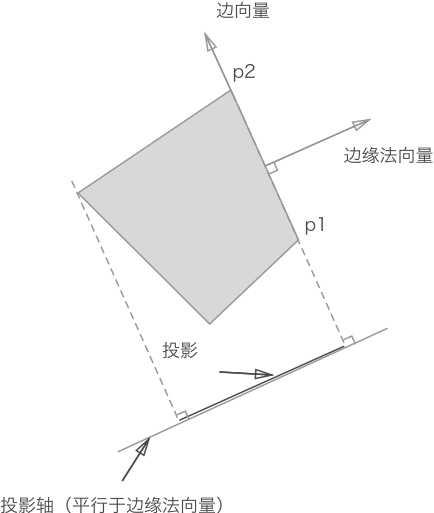``````// 以原点(0,0)为始，顶点为末。最后通过向量减法得到 边缘向量。
var v1 = new Vector(p1.x, p1.y)
v2 = new Vector(p2.x, p2.y)

// 首先得到边缘向量，然后再通过边缘向量获得相应边缘法向量（单位向量）。
// 两向量相减得到边缘向量 p2p1（注：上面应该有个右箭头，以表示向量）。
// 设向量 p2p1 为(A,B)，那么其法向量通过 x1x2+y1y2 = 0 可得：(-B,A) 或 (B,-A)。
axis = v1.edge(v2).normal()
``````

``````var Vector = function(x, y) {
this.x = x
this.y = y
}

Vector.prototype = {
// 获取向量大小（即向量的模），即两点间距离
getMagnitude: function() {
return Math.sqrt(Math.pow(this.x, 2),
Math.pow(this.y, 2))
},
// 点积的几何意义之一是：一个向量在平行于另一个向量方向上的投影的数值乘积。
// 后续将会用其计算出投影的长度
dotProduct: function(vector) {
return this.x * vector.x + this.y + vector.y
},
// 向量相减 得到边
subtarct: function(vector) {
var v = new Vector()
v.x = this.x - vector.x
v.y = this.y - vector.y
return v
},
edge: function(vector) {
return this.substract(vector)
},
// 获取当前向量的法向量（垂直）
perpendicular: function() {
var v = new Vector()
v.x = this.y
v.y = 0 - this.x
return v
},
// 获取单位向量（即向量大小为1，用于表示向量方向），一个非零向量除以它的模即可得到单位向量
normalize: function() {
var v = new Vector(0, 0)
m = this.getMagnitude()
if(m !== 0) {
v.x = this.x / m
v.y = this.y /m
}
return v
},
// 获取边缘法向量的单位向量，即投影轴
normal: function() {
var p = this.perpendicular()
return p .normalize()
}
}
``````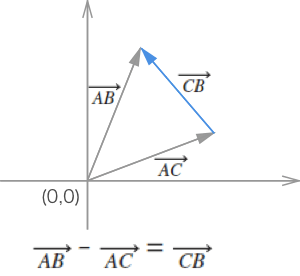### 投影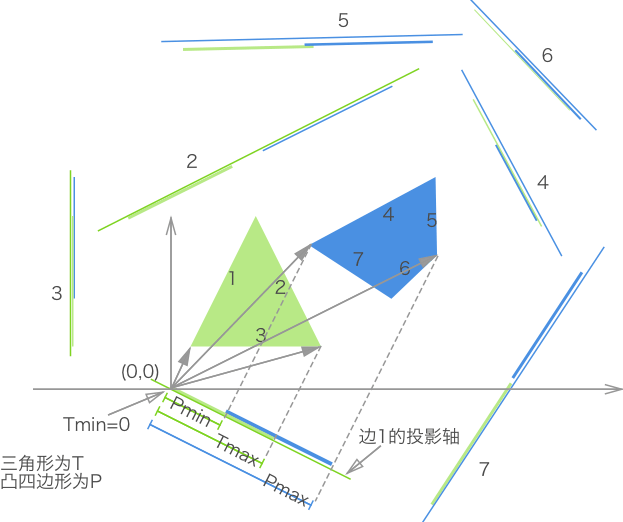``````// 用最大和最小值表示某一凸多边形在某一投影轴上的投影位置
var Projection = function (min, max) {
this.min
this.max
}

projection.prototype = {
// 判断两投影是否重叠
overlaps: function(projection) {
return this.max > projection.min && projection.max > this.min
}
}
``````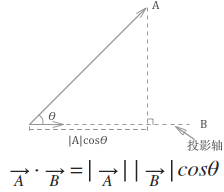``````// 根据多边形的每个定点，得到投影的最大和最小值，以表示投影。
function project = function (axis) {
var scalars = [], v = new Vector()

this.points.forEach(function (point) {
v.x = point.x
v.y = point.y
scalars.push(v.dotProduct(axis))
})
return new Projection(Math.min.apply(Math, scalars),
Math.max,apply(Math, scalars))
}
``````

### 圆形与多边形之间的碰撞检测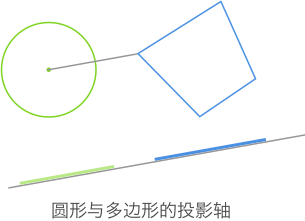See the Pen SeparatingAxisTheorem by Jc (@JChehe) on CodePen.

• 精确

• 不适用于凹多边形

• 任意凸多边形和圆形。

### 延伸：最小平移向量（MIT）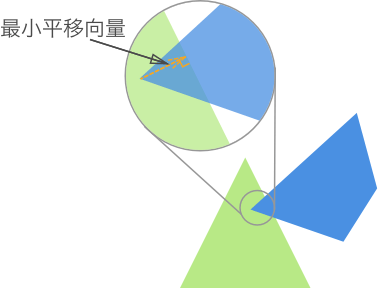# You May Also Like